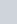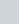If the points (2, 1) and (1, −2) are equidistant
Question:

If the points (2, 1) and (1, −2) are equidistant from the point (xy), show that x + 3y = 0.

Solution:

The distance $d$ between two points $\left(x_{1}, y_{1}\right)$ and $\left(x_{2}, y_{2}\right)$ is given by the formula

$d=\sqrt{\left(x_{1}-x_{2}\right)^{2}+\left(y_{-}-y_{2}\right)^{2}}$

Here it is said that the points (21) and (12) are equidistant from (x, y).

Letbe the distance between (21) and (x, y).

Letbe the distance between (1, −2) and (x, y).

So, using the distance formula for both these pairs of points we have

$d_{1}=\sqrt{(2-x)^{2}+(1-y)^{2}}$

$d_{2}=\sqrt{(1-x)^{2}+(-2-y)^{2}}$

Now since both these distances are given to be the same, let us equate bothand$d_{1}=d_{2}$

$\sqrt{(2-x)^{2}+(1-y)^{2}}=\sqrt{(1-x)^{2}+(-2-y)^{2}}$

Squaring on both sides we have,

$(2-x)^{2}+(1-y)^{2}=(1-x)^{2}+(-2-y)^{2}$

$4+x^{2}-4 x+1+y^{2}-2 y=1+x^{2}-2 x+4+y^{2}+4 y$

$-4 x-2 y=-2 x+4 y$

$-2 x-6 y=0$

$x+3 y=0$

Hence we have proved that when the points $(2,1)$ and $(1,-2)$ are equidistant from $(x, y)$ we have $x+3 y=0$.